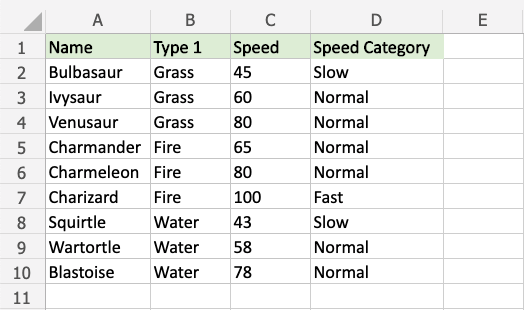# Excel IFS Function

## IFS Function

The IFS function is a premade function in Excel, which returns values based on one or more true or false conditions.

It is typed `=IFS` and has two or more parts:

=IFS(logical_test1, value_if_true1, [logical_test2, value_if_true2], [logical_test3; ...)

The conditions are referred to as `logical_test1, logical_test2, ...`, which can check things like:

• If a number is greater than another number `>`
• If a number is smaller than another number `<`
• If a number or text is equal to something `=`

Each condition is connected with a return value.

Note: More than one condition can be true so the function will return the value for the first true condition.

Note: The different parts of the function are separated by a symbol, like comma `,` or semicolon `;`

The symbol depends on your Language Settings.

## Example IFS function

Make categories for how fast the Pokemon are:

The conditions and return values are:

• Speed more than 90: "Fast"
• Speed more than 50: "Normal"
• Speed less than or equal to 50: "Slow"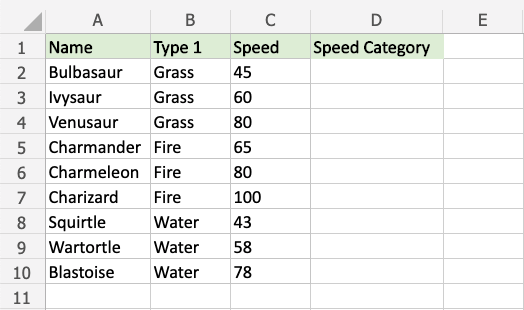Copy Values

Example IFS function, step by step:

1. Select the cell `D2`
2. Type `=IFS`
3. Double click the IFS command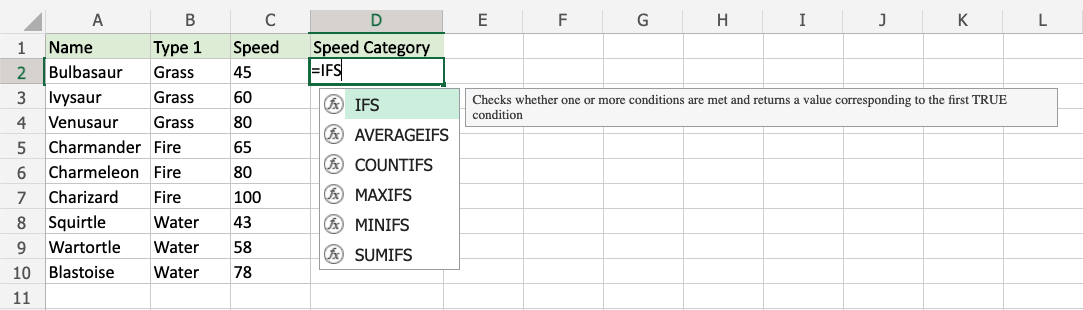1. Specify the first condition `C2>90`
2. Type `,`
3. Specify the value `"Fast"` for when the first condition is TRUE
4. Type `,`
5. Specify the second condition `C2>50`
6. Type `,`
7. Specify the value `"Normal"` for when the second condition is TRUE
8. Type `,`
9. Specify the third condition `C2<=50`
10. Type `,`
11. Specify the value `"Slow"` for when the third condition is TRUE
12. Hit enter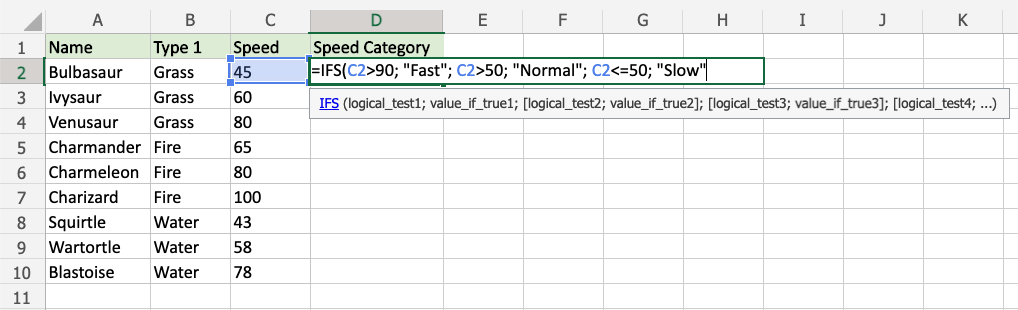Since the value in cell `C2` is "45", the first and second conditions are false, and third condition is true (less than or equal to 50), the function will return "Slow".

Note: Text values needs to be in quotes: " "

The function can be repeated with the filling function for each row to perform the same check for each Pokemon: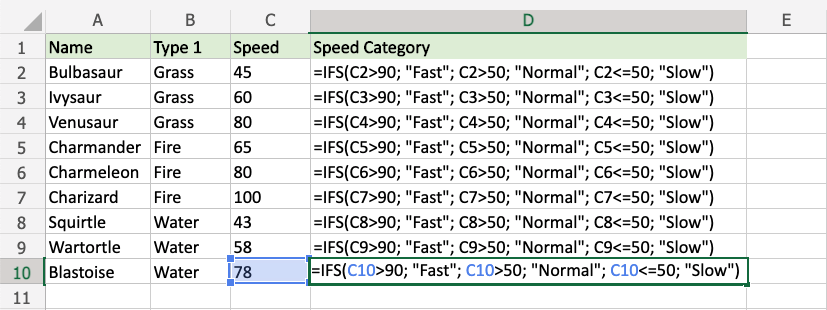Note: The third condition `<=50` includes `=` so that 50 is included in "less than or equal to 50"

Now, each Pokemon has a speed category: